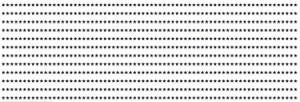Going through the Oreilly book Practical C++ Programming, which may be an arguable title, and I’m just trying to go through all of the examples and save them somewhere so I know where I left off the next time I’m feeling recklessly productive.

Exercise 4-2: Write a program to print a block E using asterisks (*), where the E is 7 characters high and 5 characters wide.

Of course the easy way would be to just print out a bunch of strings. But I consider that cheap. So I played around with struct and typedefs but I don’t know what the hell I’m doing with those so I instead forced myself to learn about passing by value vs. passing by reference.

First, the function makeE() creates an integer array which in my world of goals could be a game model, or x,y,z points for some game object. Then printE() prints out the array, but only an array of 7. If I wanted to do this whole thing much better I’d use a vector.

It’s rough shifting gears back to C++. I don’t know if I’ll ever get really good with it and be able to get to the fun stuff. You can see below that another version just printed out way too many *’s.```/*
*  4-2.cpp
*  Practical C++ Exercises
*
*  Created by Chris on 1/21/07.
*
*/
// write a program to print a block E using *, where e is 7 high and 5 wide.
#include

void makeE(int int_array[])
{
int_array=5;
int_array=1;
int_array=1;
int_array=5;
int_array=1;
int_array=1;
int_array=5;
}

void printE(int e[])
{

for (int i = 0; i < 7 ; i++)
{
for (int j=0; j<e[i]; j++)
{
std::cout << "*";
}
std::cout << "\n";
}
}

int main()
{
int e_array;

makeE(e_array);
printE(e_array);
return(0);
}
</pre>